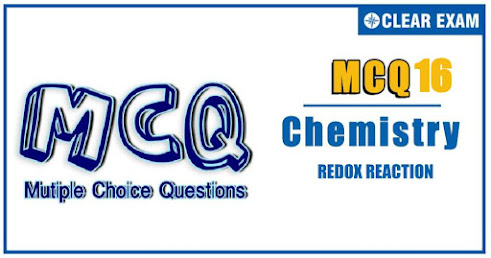## [LATEST]\$type=sticky\$show=home\$rm=0\$va=0\$count=4\$va=0

IIT JEE exam which consists of JEE Main and JEE Advanced is one of the most important entrance exams for engineering aspirants. The exam is held for candidates who are aspiring to pursue a career in the field of engineering and technical studies. Chemistry is important because everything you do is chemistry! Even your body is made of chemicals. Chemical reactions occur when you breathe, eat, or just sit there reading. All matter is made of chemicals, so the importance of chemistry is that it's the study of everything..

Q1. The oxidation states of the most electronegative elements in the products of the reaction between BaO2 and H2SO4 are
•  0 and -1
•  -1 and -2
•  -2 and 0
•  -2 and +1
Solution
(b). BaO_2+H_2 SO_4 (dil)⟶BaSO_4+H_2 O_2 Oxidation state of O in H2O2=-1 Oxidation state of O in BaSO4=-2

Q3. , The number of peroxide bond in perxenate ion[XeO_6 ]^(4-)is
•   0
•  2
•  3
•  1
Solution
(a). No Peroxide bond in [XeO_6 ]^(4-).

Q4. The number of moles of K2 Cr2O7 reduced by 1 mol of Sn^(2+)ions is
•  1/3
•  3
•  1/6
•  6
Solution
(a). (6e^-+Cr2O7^(2-)⟶2Cr^(3+)) Sn^(2+)⟶Sn^(4+)+2e^- Equivalent of Cr2O7^(2-)=Equivalent of Sn^2^+ (n=6) (n=2) ⇒1 Eq=1 Eq 1/6 mol=1/2 mol 1/3 mol of Cr2O7^(2-)=1 mol of Sn^(2+)

•  (CH3)2-CH-OH
•  (CH3)2CH-N=N-Br
•  (CH3)2C(NO2)-N=O
•  (CH3)2C-NHOH
Solution
(c). The 2° (RNO2) group reacts with HNO2 (2NaNO2+H2SO4→2HNO2+2Na2SO4 )to give pseudonitrole, which gives blue colour with NaOH

Solution option-(a)
Q7.In the reaction 3Cl2+6NaOH⟶NaClO3+5NaCl+3H2O the element which loses as well as gains electrons is
•  Na
•  O
•  Cl
•  None of these
Solution
(c) It is a disproportionation reaction, so Cl2 undergoes both oxidation and reduction.

•  Wolff –Kishner reduction
•  Clemmensen reduction
•  LAH
•  NaBH4
Solution
(a). Wolff–Kishner reduction converts group to (-CH2) group without dehydrating alcohols to alkene. So the answer is (a)

Q9.In the reaction 8Al+3Fe_3 O_4⟶4Al_2 O_3+9Fe The number of electrons transferred from the reductant to the oxidant is
•  8
•  4
•  16
•  24
Solution
(d). 8Al⟶8Al^(3+)+24e^- 9Fe^(8/3+)+24e^-⟶9Fe
Q10. In the neutralization of Na2S2O3 using K2 Cr2O7 by iodometry, the equivalent weight of K2 Cr2O7 is
•  M/2
•  M/6
•  M/3
• M
Solution
(b) 6e^-+Cr2O7^(2-)⟶2Cr^(3+) (n=6) 2S2O3^(2-)⟶S4O6^(2-)+2e^- (n=2) ∴ Equivalent weight ofK2Cr2O7=M/6#### Written by: AUTHORNAME

AUTHORDESCRIPTION## Want to know more

Please fill in the details below:

## Latest NEET Articles\$type=three\$c=3\$author=hide\$comment=hide\$rm=hide\$date=hide\$snippet=hide

Name

ltr
item
BEST NEET COACHING CENTER | BEST IIT JEE COACHING INSTITUTE | BEST NEET & IIT JEE COACHING: REDOX REACTIONS Quiz-16
REDOX REACTIONS Quiz-16